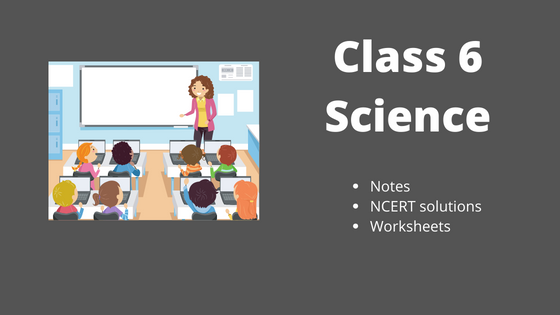# NCERT Solutions for class 6 Science, Notes, Worksheets

•• Welcome to our class 6 Science page. In this page you can find class 6Science ncert solutions , notes, assignments, worksheets etc. You can share the link to free Class 6 NCERT Science solutions with your classmates as all study material is free of cost.

1. First read and understand the notes.
2. Try to go through the solved examples of the chapter you are reading
3. Now it is the time to solve unsolved assignments and worksheets. You will find plenty of them here or on the respective chapter page.

## Frequently asked Questions on CBSE Class 6 Science

### How many chapters are there in Class 6 Science?

There are total 11 chapters in Class 6 Science as per the rationalized Syllabus. All the chapters are required for assessment

### What is the best books for Class 6 Science?

Couple of book
(a)NCERT Class 6 Maths textbook
(b)NCERT Class 6 Exemplar
(c)Lakhbir singh

### Which is the easiest chapter of class 6 Science NCERT Text book?

Components of Food and Sorting material are the easiest chapter

### Is Class 6 Science NCERT Text book Sufficient for CBSE Exams?

It is sufficient if you go through all the questions in the NCERT text book thoroughly and practice them all.However to enhance your maths skill , you can go for NCERT exemplar and other books

### what is the best way to learn the concept in Class 6 Science?

First go through all the Notes and solved examples and Then do the exercises. Practice Lot of questions. The simple Mantra is Practice .Practice and Practice

### what is the deleted syllabus in Class 6 Science?

Chapter Food:Where does it come from : Full chapter deleted
Chapter Fibre to Fabric: Full chapter deleted
Chapter Changes around us: Full chapter deleted
Chapter Water: Full chapter deleted
Chapter Garbage In ,Garbage out: Full chapter deleted### Practice Question

Question 1 What is $\frac {1}{2} + \frac {3}{4}$ ?
A)$\frac {5}{4}$
B)$\frac {1}{4}$
C)$1$
D)$\frac {4}{5}$
Question 2 Pinhole camera produces an ?
A)An erect and small image
B)an Inverted and small image
C)An inverted and enlarged image
D)None of the above